# Estimated seasonal cycles

This map below shows the annual and semi-annual cycles fitted to the longest available window of data at each PSMSL station with 70% of annual data present. These cycles were estimated as part of our trend fitting procedure.

The results for each cycle are presented here as coefficients of the sine wave $h = A \sin (\omega t + \phi)$ where $$t$$ is the time in years, such that the fractional part of $$t$$ is zero on midnight at the start of 1st January,
$$\omega$$ is $$2\pi$$ for the annual cycle, and $$4\pi$$ for the semi-annual cycle,
$$A$$ is the amplitude of the sine wave, given in metres
$$\phi$$ is the phase shift, given here in degrees

Select desired plot: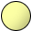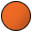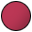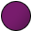< 20 20 to 40 40 to 60 60 to 80 80 to 100 100 to 120 > 120 Amplitude of seasonal cycle, mm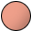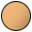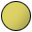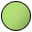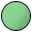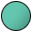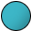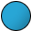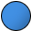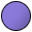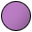January February March April May June July August September October November December Peak month of annual cycleJanuary & July February & August March & September April & October May & November June & December Peak months of semi-annual cycle